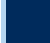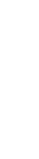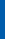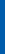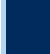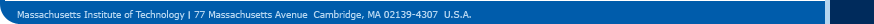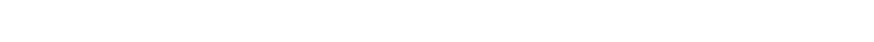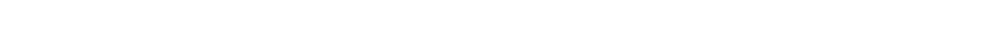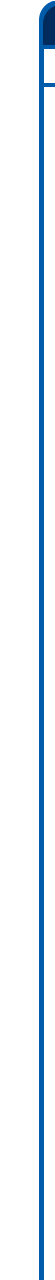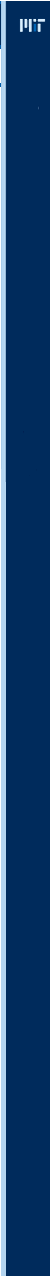6.435 - Theory of Learning and System Identification - Spring 2007Description Syllabus References Homeworks Scribing Project TopicsGiven a finite set of Data: { x_1, x_2, ..., x_n, y_1, y_2, ..., y_n}, how can we derive the functional dependence in this data in terms of a "dynamic" model that is both parsimonious and predictive? The answer to this question is quite elusive unless we add more assumptions on "how" the data is generated. Many disciplines address various sets of assumptions on the data. If the pairs (x_i, y_i ) are assumed probabilistic and IID, then statistical learning theory addresses the above question through the notion of Risk Minimization, which can be viewed as a generalization of the problem of density estimation. Within this theory, the functional dependence is hypothesized through the selection of a class of functions, whose dimension in addition to a notion of Empirical Risk Minimization, control the predictive power of the derived model. If the data set is dependent, then time-series analysis using linear models offers a powerful paradigm for deriving a predictive model from data. The theory of Hidden Markov Models also plays an important role under this assumption. In all such cases, there is always the question of model selection. Notions related to "Description Length" and "Kolmogorov Complexity" play a central role in answering such a question. In this course, we present a unified framework that addresses the above problem. We demonstrate that the notion of Empirical-Risk Minimization encompasses a variety of standard statistical inference problems including Function Approximation, Regression Analysis, Density Estimation, and Prediction-Error Minimization. We show that the notion of Uniform Convergence of Empirical Risk is central to all such problems. We derive conditions for such convergence in terms of the Complexity of the model set. In particular, we derive: finite-data bounds on the difference between Empirical Risk and actual Risk in terms of the data length and the complexity of the model set known as VC dimension, asymptotic bounds and asymptotic distribution of estimated parameters in the case of linear models and Hidden Markov Models for which we bring out the role of Relative Entropy and the notion of input selection and persistence of excitation, a complexity notion that can be used to evaluate model sets known as Description Length and its relationship to Structured Risk Minimization, algorithms for constructing such models including Support Vector Machines, Least Squares, Maximum Likelihood, and Expectation Minimization, and tools for experiment design. While the course presents a rigorous treatment of this subject, most proofs will be done for Indicator Functions in the case of independent Data (which emerge in applications in Pattern Recognition), and Guass-Markov Models and Hidden Markov Models in the case of dependent data. Hidden Markov Fields and Graphical Models will also be addressed.